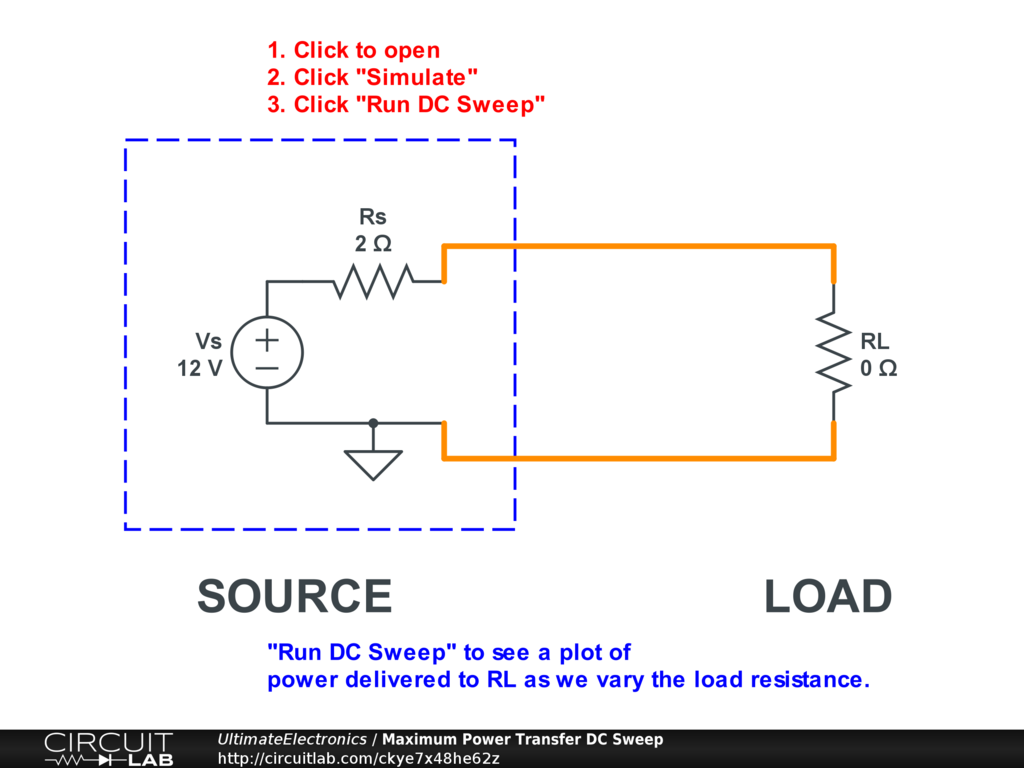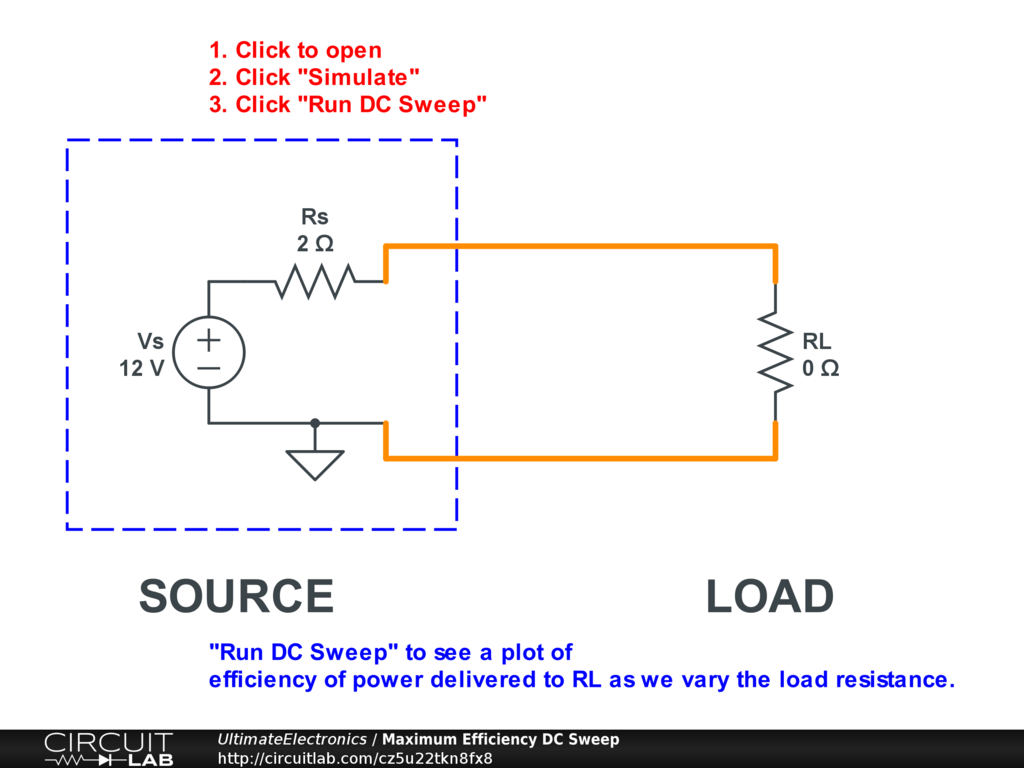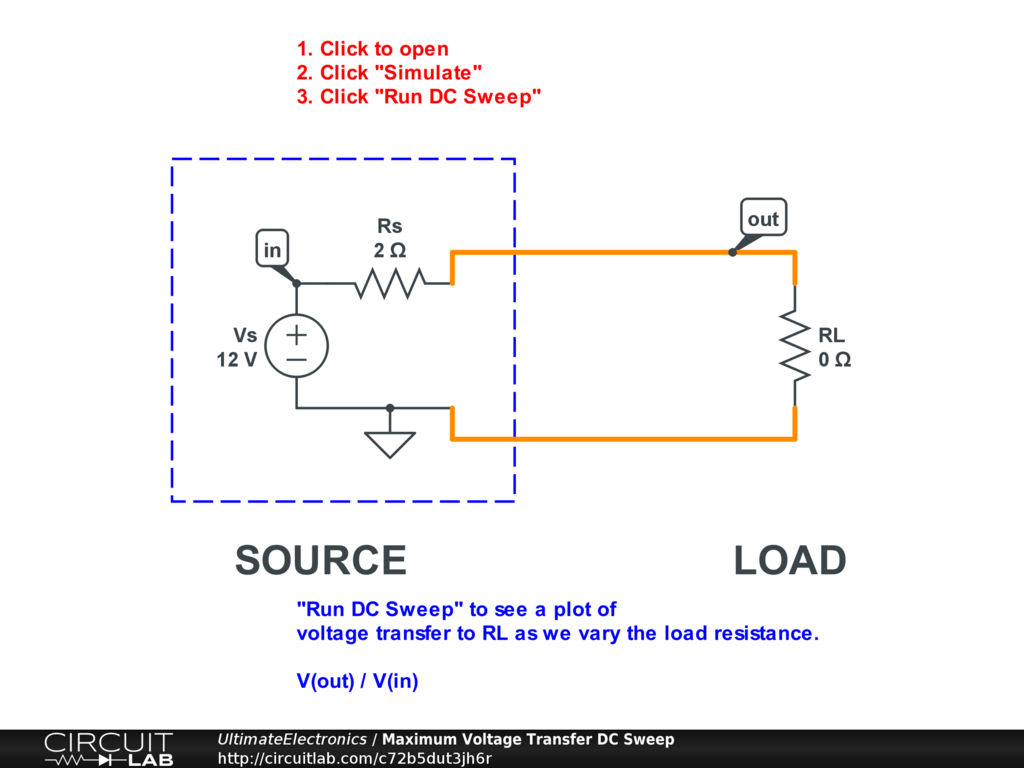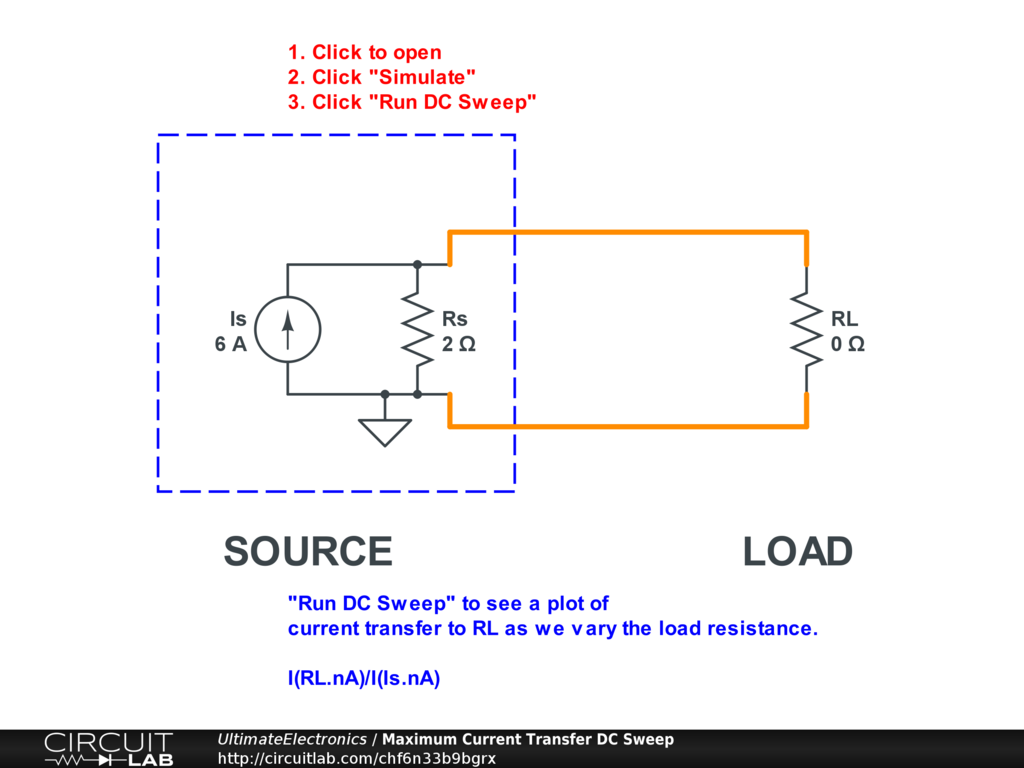Maximum Power Transfer and Impedance Matching

In the previous section, Thevenin Equivalent and Norton Equivalent Circuits, we discussed how we could simplify complicated linear networks into very simple ones involving a single source and a single resistor.

We can use this very simple Thevenin equivalent circuit model to understand how power or signal is transferred between blocks of a circuit when we model each block's input or output as an equivalent circuit.

(See a review of power in electrical circuits.)

Maximum Power Transfer

Suppose we have a voltage source $V_S$ with a known, fixed, nonzero series source resistance $R_S > 0$. It's connected to a load which we're modeling as just a resistor.

For what value of load resistor will we transfer the most power to the load?

Here's a circuit simulation that shows a numerical example:Interactive Exercise Click the circuit, then click "Simulate," and "Run DC Sweep" to see a plot of the power delivered to the load $\text{P(RL)}$ as we vary the resistance of RL.

This is a very linear circuit, but the curve produced here is quite nonlinear.

We can solve this simple circuit by hand. The voltage source pushes current through a total resistance $R_S + R_L$, so the total current is:

$$I = \frac {V_S} {R_S + R_L}$$

The power delivered to the resistor $R_L$ is $I^2 R_L$, or:

\begin{align} \text{P(RL)} & = \left( \frac {V_S} {R_S + R_L} \right) ^2 R_L \\ & = V_S^2 \frac {R_L} {(R_S + R_L)^2} \end{align}

If $V_S$ and $R_S$ are fixed, then we're only looking at the behavior of the terms of the fraction involving $R_L$.

Look at the limits here:

1. Load is short circuit. If $R_L = 0$, then the numerator goes to zero, so $\text{P(RL)} = 0$. No power is delivered if the load is a short circuit.
2. Load is open circuit. If $R_L = \infty$, then the numerator and denominator both go to infinity, but the denomniator has a squared term so it goes to infinity faster. (See Algebraic Approximations for more details.) The result is that the fraction goes to 0, and $\text{P(RL)} = 0$. No power is delivered if the load is an open circuit.
3. Load is intermediate. If our load resistance is somewhere $0 < R_L < \infty$, then there'll be some nonzero power $\text{P(RL)} > 0$.

We can use calculus to find the maximum by solving for the value of $R_L$ that makes the derivative $\frac {d P(RL)} {d R_L} = 0$. (Do this as an exercise!)

The result is that the maximum power occurs if $R_L = R_S$.

At this maximum power point, the power transferred is:

\begin{align} \text{P(RL)}_{\text{max}} & = V_S^2 \frac {R_S} {(R_S + R_S)^2} \\ & = V_S^2 \frac {R_S} {(2 R_S)^2} \\ & = \frac {V_S^2} {4 R_S} \end{align}

At this maximum power point, the same amount of power is dissipated in the load resistor and the source resistor:

$$\text{P(VS)} = \text{P(RS)} + \text{P(RL)} = \frac {V_S^2} {2 R_S}$$

Note that this is the same as the power supplied by a voltage source connected to a single equivalent resistor of $R_{\text{eq}} = 2 R_S$.

Maximum Efficiency

The maximum power point is not the same as the maximum efficiency point. For review, we discussed efficiency previously in our sections on Power and Thermodynamics, Energy, and Equilbrium.

The efficiency here is defined as the ratio of power delivered to the load divided by power supplied by the source:

\begin{align} \eta & = \left| \frac{P(RL)} {P(VS)} \right| \\ & = \frac { V_S^2 \frac {R_L} {(R_S + R_L)^2} } { V_S I } \\ & = \frac { V_S R_L } { (R_S + R_L)^2 \frac {V_S} {R_S + R_L} } \\ & = \frac { R_L } { R_S + R_L } \end{align}

That's it.

1. Load is short circuit. If $R_L = 0$, then the efficiency $\eta = 0$.
2. Load is open circuit. If $R_L = \infty$, then the efficiency $\eta = 1$.
3. Load is maximum power point. If $R_L = R_S$ then the efficiency $\eta = 0.5$.

We can modify our simulation to plot efficency rather than just maximum power:Interactive Exercise Click the circuit, click "Simulate," and "Run DC Sweep" to plot the efficiency as we vary the load resistance.

When we plug an appliance into a wall outlet, we're not interested in maximum power transfer, but in good-enough efficency. If our electric microwave oven consumes 1500 W of power, it'd be really bad if we also had 1500 W of heat dissipation within the walls of our house!

Maximum Signal Transfer

When interconnecting parts of an electronic system, such as amplifer, we may not be interested in power transfer, but instead just signal transfer. Signal can be carried either as voltage or as current (or as power, in which case maximum power transfer does apply!).

Maximizing the voltage transfer ratio make sense from a perspective of a voltage source:Interactive Exercise Click the circuit, click "Simulate," and "Run DC Sweep" to see how the voltage delivered to the load varies as we adjust the load resistance. If you run the simulation, you'll see clearly that the maximum voltage transfer occurs when $R_L \gg R_S$.

Maximizing the current transfer ratio makes sense if we transform the source from a Thevenin equivalent to a Norton equivalent circuit:Interactive Exercise Click the circuit, click "Simulate," and "Run DC Sweep" to see how the voltage delivered to the load varies as we adjust the load resistance. If you run the simulation, you'll see clearly that the maximum current transfer occurs when $R_L \ll R_S$.

Impedance Matching

In Complex Numbers we started to explore how components other than resistors can present themselves as imaginary resistance values.

When we start working in the frequency domain and including capacitors and inductors in our circuits, then the idea of "resistance matching" for maximum power transfer becomes broadened to "impedance matching," where impedance is essentially a complex-valued resistance.

In these cases, the maximum power transfer point is for the source impedance $Z_S$ and the load impedance $Z_L$ to be complex conjugates. As an exercise, you can write the source impedance $Z_S = a + b j$ and the load impedance $Z_L = c + d j$ and solve the maximum power equations above. You'll find that the maximum power delivered to the load happens with $a = c$ and $b = - d$.

Deciding What to Maximize

In design problems, these are merely theoretical tools to think about the extremes of matching various sources and loads.

The assumption made throughout this section is that the source resistance $R_S$ is fixed. In many design problems, this is not the case. However, it is nice to quickly see that if we have a design with a particular source resistance, then we do have an upper bound on our power output.

A few guidelines for building intuition about what to maximize:

• In many design problems particularly with small signals or RF, minimizing noise is more important that maximizing power or signal transfer. This may or may not coincide with impedance matching.
• In many problems with transmission lines or antennas, impedance matching is more important because of minimizing reflections and preserving the integrity of the signal than for pure power transfer purposes. In these high-frequency applications, the lumped element model no longer applies, the speed of light matters, and the system can no longer be assumed to be at equilibrium instantaneously.
• In many design problems internal to a circuit, minimizing inter-stage loading is more important than impedance matching or power transfer. In these cases we want to ensure good voltage transfer or good current transfer, depending on how our signal is represented.

What's Next

In the next section, Dependent (Controlled) Sources, we'll add several new types of voltage and current sources that are invaluable for modeling more complicated components.